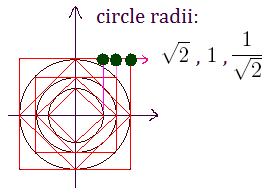## Sunday, May 2, 2010

### M Theory Lesson 322

Observe that the choice of coefficients $(r,1)$ in a scaled $R_2$ factoris the general case for a super magic circulant, because scale factors will always be absorbed into the normalisation. Under an interchange of the real and complex parameters in $M$, the row sums and norm square sums remain unaltered. This means that $(r,1)$ is equivalent to $(r^{-1},1)$, a kind of S duality. For example, in the tribimaximal case it doesn't matter if we use $\sqrt{2}$ or $\sqrt{2}^{-1}$. This is an inversion of parameters with respect to the unit circle, as inThus magic circulants, although very simple, provide an interesting parameter space in which to study mixing matrices. Note that the inverse of an $R_2$ factor is given by sending $i$ to $-i$, so we might think of the (charged lepton) identity mixing matrix as two inverse $R_2$ factors with parameters $a = r$ and $b = -r$. This gives three parameters in total for lepton mixing.

#### Post a Comment

Note: Only a member of this blog may post a comment.